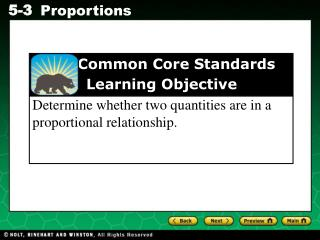DownloadDownload PresentationCommon Core Standards Learning Objective

# Common Core Standards Learning Objective

Télécharger la présentation## Common Core Standards Learning Objective

- - - - - - - - - - - - - - - - - - - - - - - - - - - E N D - - - - - - - - - - - - - - - - - - - - - - - - - - -
##### Presentation Transcript

1. Common Core Standards Learning Objective Determine whether two quantities are in a proportional relationship.

2. Vocabulary proportion cross products

3. 2 3 2 3 4 6 4 6 = An equation that states that two ratios are equivalent is called a proportion. For example, the equation, or proportion, states that the ratios and are equivalent. Ratios that are equivalent are said to be proportional, or in proportion.

4. In the proportion , the products a ∙ d and b ∙ c are called cross products. a b a b c d c d = = Proportion a∙ d = b ∙ c Cross Products One way to find whether two ratios are equivalent is to find their cross products.

5. 6 15 6 15 4 10 4 10 ? ? = = ? 6  10 = 4  15 Additional Example 1A: Using Cross Products to Identify Proportions Tell whether the ratios are proportional. Find the cross products. 60 = 60 Since the cross products are equal, the ratios are proportional.

6. 4 1 15 5 ? 4 parts gasoline 1 part oil ? 15 quarts gasoline 5 quarts oil = = ? 4  5 = 1  15 Additional Example 1B: Using Cross Products to Identify Proportions A mixture of fuel for a certain small engine should be 4 parts gasoline to 1 part oil. If you combine 5 quarts of oil with 15 quarts of gasoline, will the mixture be correct? Set up equal ratios. Find the cross products. 20  15 The ratios are not equal. The mixture will not be correct.

7. 5 10 5 10 2 4 2 4 ? ? = = ? 5  4 = 2  10 Check It Out! Example 1A Tell whether the ratios are proportional. Find the cross products. 20 = 20 Since the cross products are equal, the ratios are proportional.

8. 3 1 12 4 ? 3 parts tea 1 part sugar ? 12 tablespoons tea 4 tablespoons sugar = = ? 3  4 = 1  12 Check It Out! Example 1B A mixture for a certain brand of tea should be 3 parts tea to 1 part sugar. If you combine 4 tablespoons of sugar with 12 tablespoons of tea, will the mixture be correct? Set up equal ratios. Find the cross products. 12 = 12 The ratios are equal. The mixture will be correct.

9. x 36 1 3 = 1 3 x 36 (36) = (36) Additional Example 2: Using Properties of Equality to Solve Proportions The ratio of the length of the actual height of a person to the length of the shadow cast by the person is 1:3. At the same time, a lighthouse casts a shadow that is 36 meters long. What should the height of the lighthouse be? 1 3 Write a ratio comparing height of a person to shadow length. height of person length of shadow Set up the proportion. Let x represent the shadow length. Since x is divided by 36, multiply both sides of the equation by 36. 12= x The length of the lighthouse’s shadow should be 12 meters.

10. x 20 1 5 = 1 5 x 20 (20) = (20) Check It Out! Example 2 For most cats, the ratio of the length of their head to their total body length is 1:5. If a cat is 20 inches in length, what should the total length of their head be? 1 5 Write a ratio comparing head length to total length. head length total length Set up the proportion. Let x represent the length of the cat's head. Since x is divided by 20, multiply both sides of the equation by 20. 4= x The length of the cat's head should be 4 inches.

11. 48 42 40 15 3 4 16 14 ? ? = = Lesson Quiz: Part I Tell whether the ratios are proportional. yes 1. 2. no 3. The ratio of violins to violas in an orchestra is 5:3. t The orchestra has 9 viola players. How many t violinists are in the orchestra? 15 4. Two weights are balanced on a fulcrum. If a 6 lb weight is positioned 1.5 ft from the fulcrum, at what distance from the fulcrum must an 18 lb weight be placed to keep the weights balanced? 0.5 ft

12. Java Book is comprised of two sections Theory and Practice in a ratio of 7:2. How much of each type of content will be needed to make a book of 450 pages? Theory = 350 Practice = 100 30 girls and boys are planned for a picnic. There are in a ratio of 3 girls to 7 boys. How many boys are there? 70 boys

13. Even More Questions . . . Proportions 1

14. Classwork & Homework Lesson 5-3 Practice B (all) Lesson 5-3 Problem Solving (all)